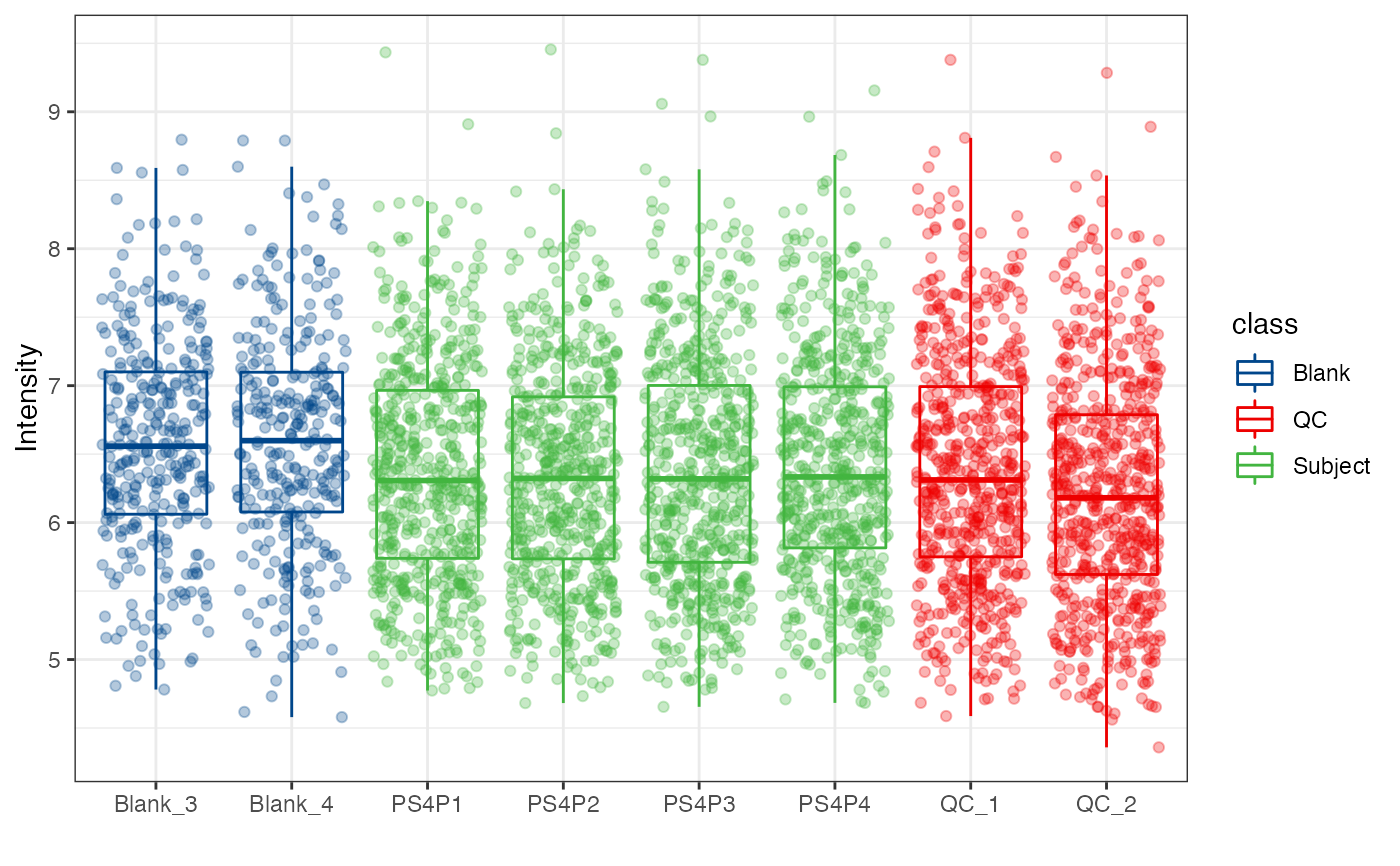Boxplot for each samples.

massqc_sample_boxplot(
object,
color_by,
fill_by,
order_by = "sample_id",
outlier.shape = NA,
point = FALSE,
point_alpha = 0.8
)

## Arguments

object

tidymass-class object.

color_by

which column (sample_info) is used to color samples

fill_by

which column (sample_info) is used to fill samples

order_by

which column (sample_info) is used to order samples

outlier.shape

outlier.shape from ggplot2.

point

point or not.

point_alpha

point_alpha

ggplot2 plot.

## Author

Xiaotao Shen shenxt1990@163.com

## Examples

library(massdataset)
library(ggplot2)
data("expression_data")
data("sample_info")
data("variable_info")

object =
create_mass_dataset(
expression_data = expression_data,
sample_info = sample_info,
variable_info = variable_info
)

object %>%
massqc_sample_boxplot()
#> Warning: NA will be removed in the boxplot.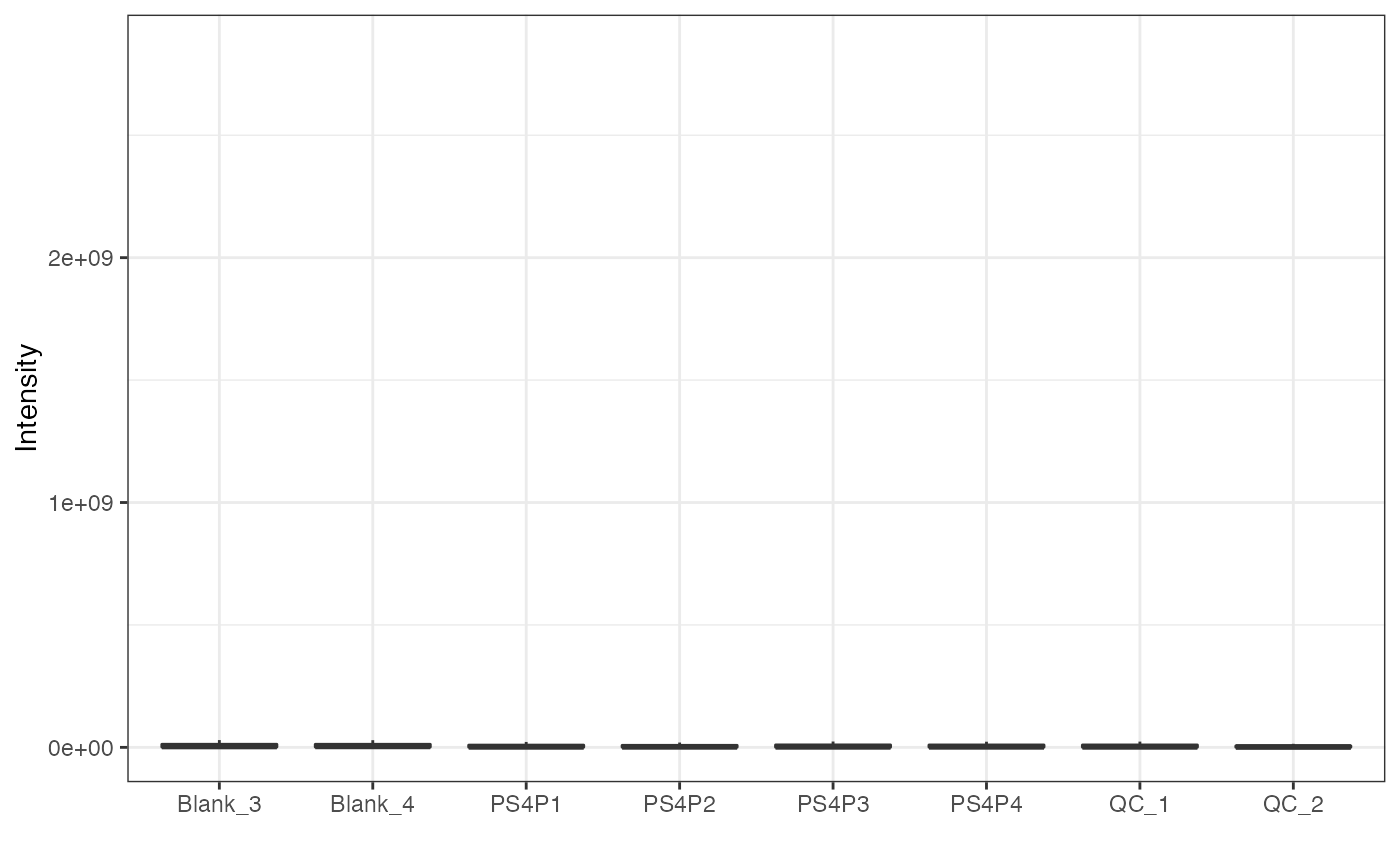object %>%
log(10) %>%
massqc_sample_boxplot()
#> Warning: NA will be removed in the boxplot.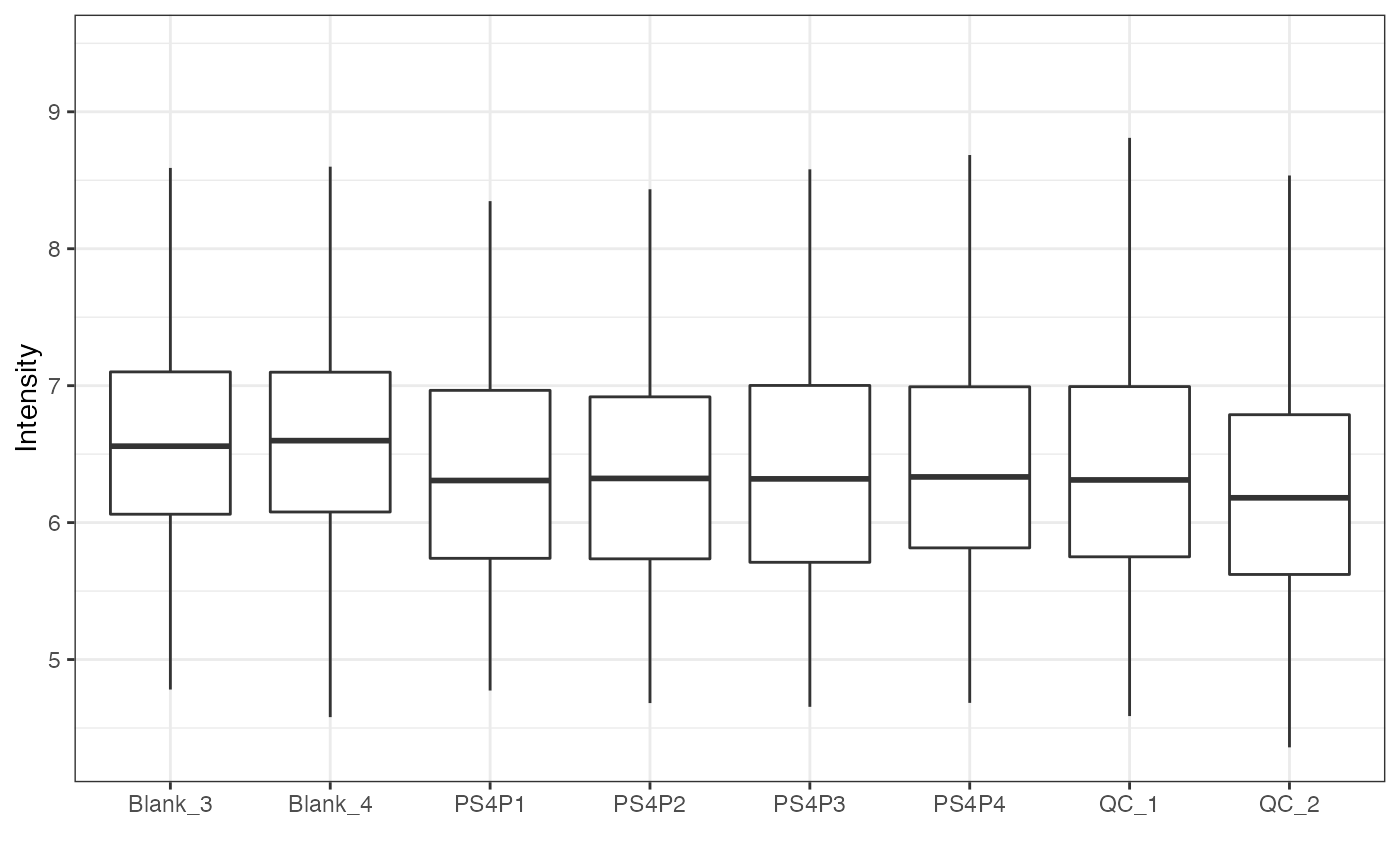object %>%
log(10) %>%
massqc_sample_boxplot(color_by = "class")
#> Warning: NA will be removed in the boxplot.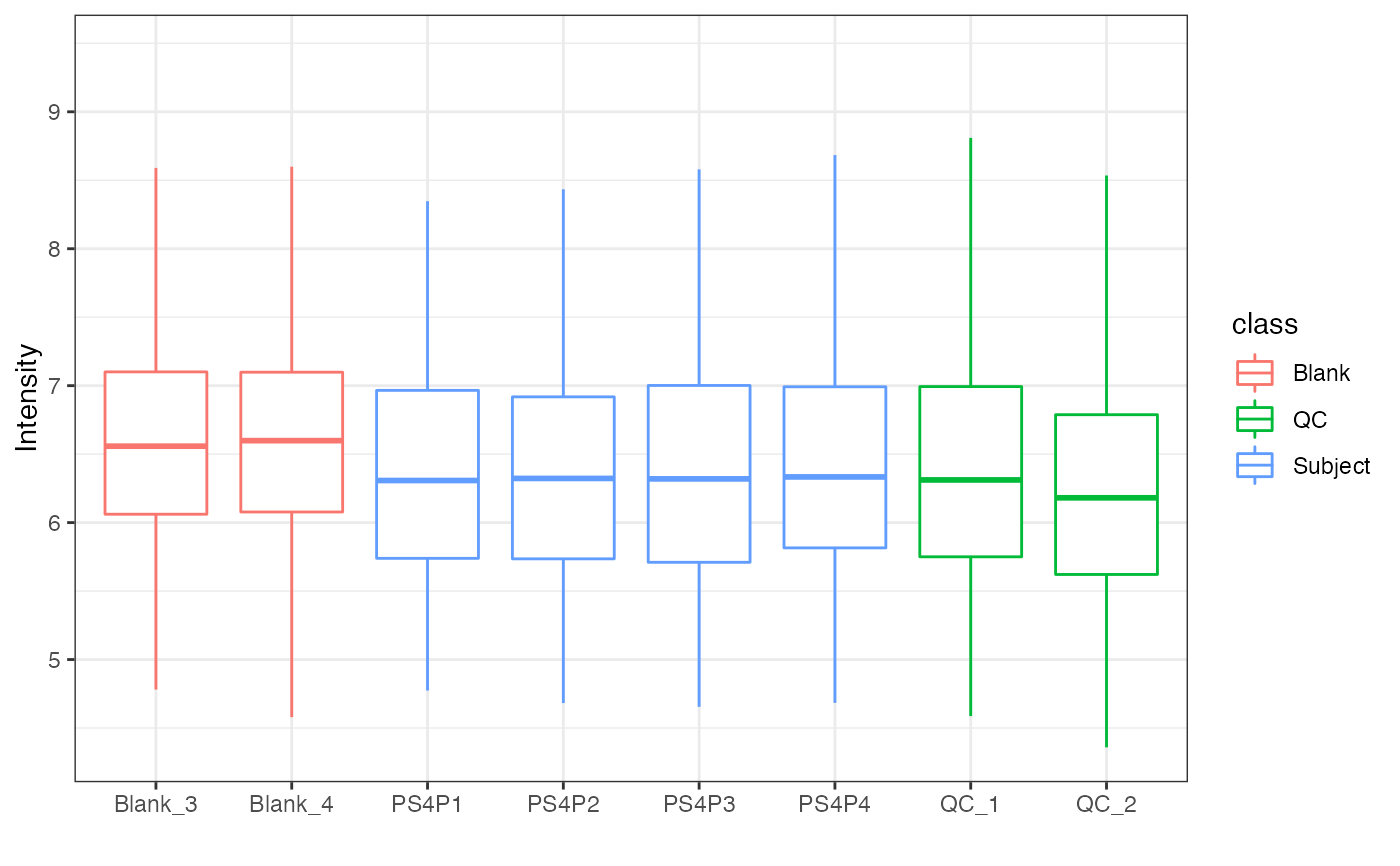object %>%
log(10) %>%
massqc_sample_boxplot(fill_by = "class") +
ggsci::scale_fill_lancet()
#> Warning: NA will be removed in the boxplot.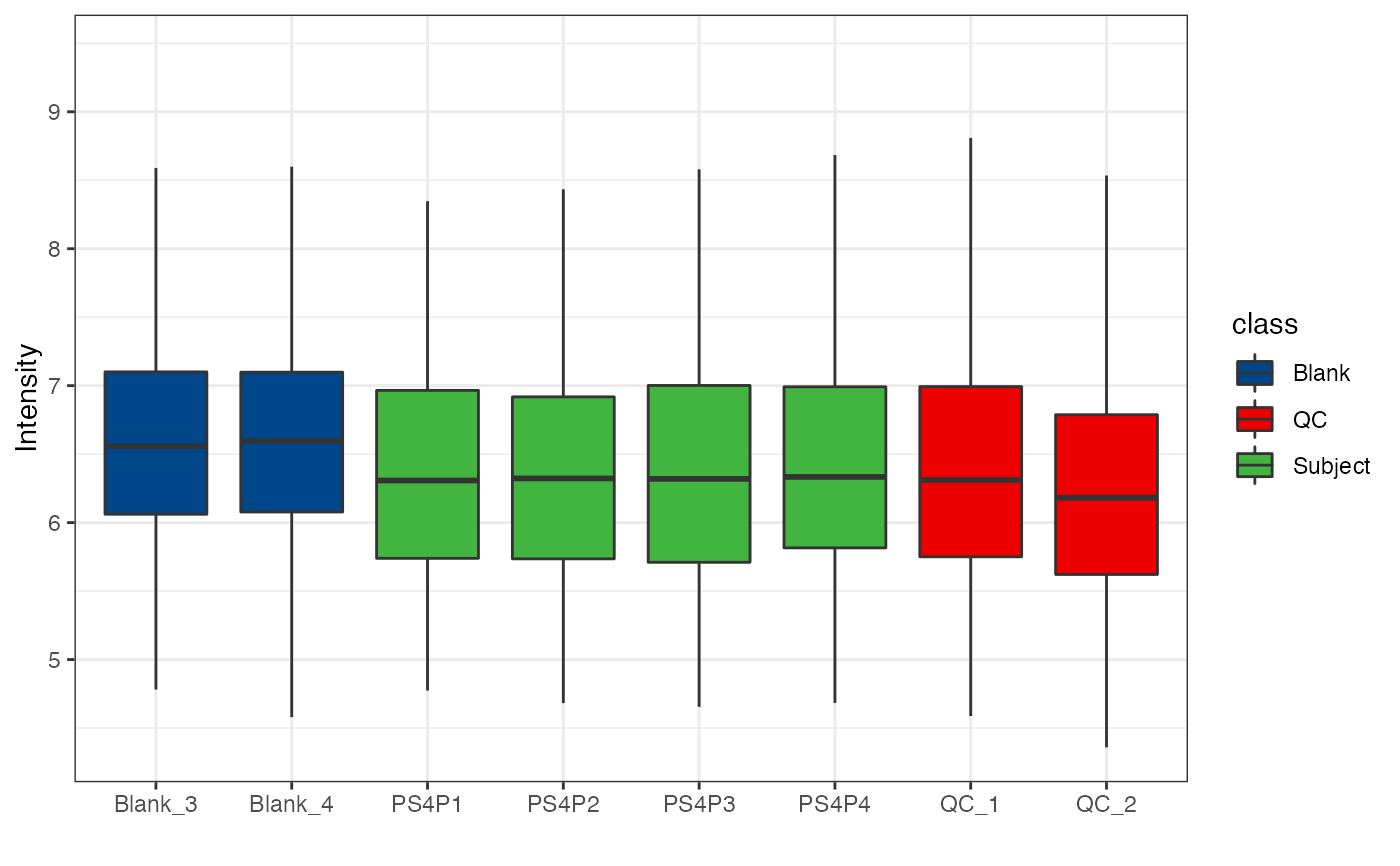object %>%
log(10) %>%
massqc_sample_boxplot(
fill_by = "class",
color_by = "class",
point = TRUE,
point_alpha = 0.3
) +
ggsci::scale_fill_lancet()
#> Warning: NA will be removed in the boxplot.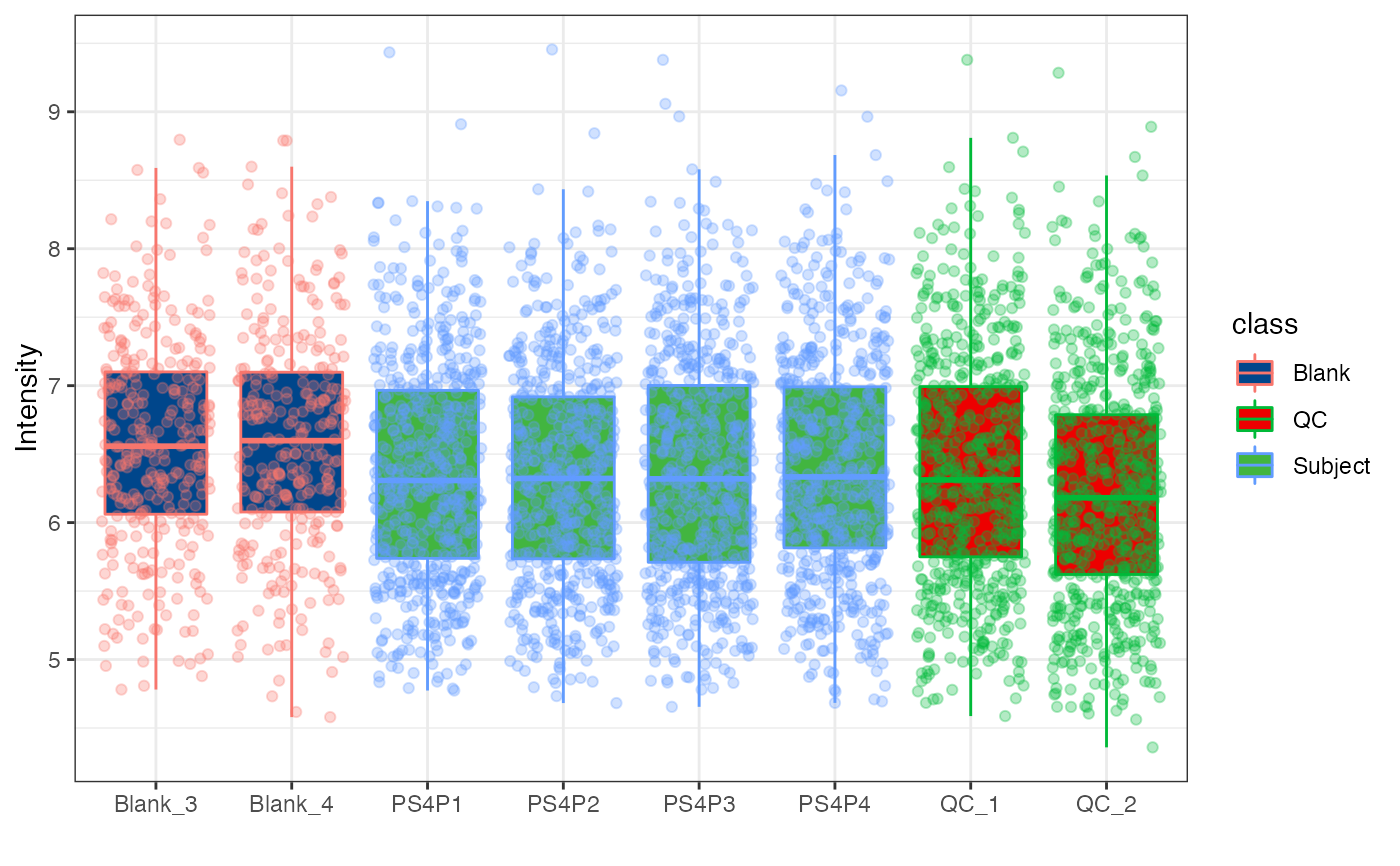object %>%
log(10) %>%
massqc_sample_boxplot(color_by = "class",
point = TRUE,
point_alpha = 0.3) +
ggsci::scale_color_lancet()
#> Warning: NA will be removed in the boxplot.